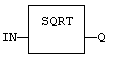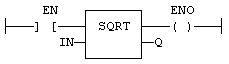# SQRT / SQRTL

Function - Calculates the square root of the input.

## Inputs

IN : REAL/LREAL  Real value

## Outputs

Q : REAL/LREAL   Result: square root of IN

## Remarks

In LD language, the operation is executed only if the input rung (EN) is TRUE. The output rung (ENO) keeps the same value as the input rung. In IL, the input must be loaded in the current result before calling the function.

Q := SQRT (IN);

## FBD Language## LD Language

(* The function is executed only if EN is TRUE *)
(* ENO keeps the same value as EN *)## IL Language:

Op1: LD  IN
SQRT
ST  Q    (* Q is: SQRT (IN) *)